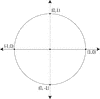# Unit Circle Labeled With Quadrantal ValuesIllustration of a unit circle (circle with a radius of 1) superimposed on the coordinate plane. At each quadrantal angle, the coordinates are given, but not the angle measure. These coordinates can be used to find the six trigonometric values/ratios. The x-coordinate is the value of cosine at the given angle and the y-coordinate is the value of sine. From those ratios, the other 4 trigonometric values can be calculated.

Unit Circles

### Source

Florida Center for Instructional Technology Clipart ETC (Tampa: University of South Florida, 2007)

EPS (vector)

354.8 KiB

TIFF (full resolution)

3017×3000, 146.7 KiB

Large GIF

1024×1018, 20.0 KiB

Medium GIF

640×636, 11.3 KiB

Small GIF

320×318, 5.3 KiB